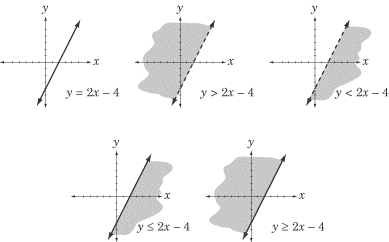# SAT数学分析：Graphing Linear Inequalities

来源：新东方在线    要点：SAT数学分析

The graph of an inequality is a graph of a region rather than a simple graph of a line. An inequality is actually the graph of all the points on the coordinate plane that are either greater or less than a particular line. For this reason, the graph of an inequality looks similar to the graph of a line but has two major differences. First, the region on one side of the line (which side depends on the inequality) is shaded. Second, the line itself is either dotted or solid depending on whether the inequality is inclusive.To summarize the above graphs: when the inequality is “greater than or equal to” or “less than or equal to,” the line in the graph is solid; when the inequality is “greater than” or “less than,” the line in the graph is dotted. Any point that satisfies the inequality lies in the shaded region, and any point that does not lies in the un-shaded region.That’s all you need to know about graphing inequalities for the Math IC.